# stepinfo

Rise time, settling time, and other step-response characteristics

## Syntax

``S = stepinfo(sys)``
``S = stepinfo(y,t)``
``S = stepinfo(y,t,yfinal)``
``S = stepinfo(y,t,yfinal,yinit)``
``S = stepinfo(___,'SettlingTimeThreshold',ST)``
``S = stepinfo(___,'RiseTimeLimits',RT)``

## Description

`stepinfo` lets you compute step-response characteristics for a dynamic system model or for an array of step-response data. For a step response y(t), `stepinfo` computes characteristics relative to yinit and yfinal, where yinit is the initial offset, that is, the value before the step is applied, and yfinal is the steady-state value of the response. These values depend on the syntax you use.

• For a dynamic system model `sys`, `stepinfo` uses yinit = 0 and yfinal = steady-state value.

• For an array of step-response data `[y,t]`, `stepinfo` uses yinit = 0 and yfinal = last sample value of `y`, unless you explicitly specify these values.

For more information on how `stepinfo` computes the step-response characteristics, see Algorithms.

The following figure illustrates some of the characteristics `stepinfo` computes for a step response. For this response, assume that y(t) = 0 for t < 0, so yinit = 0.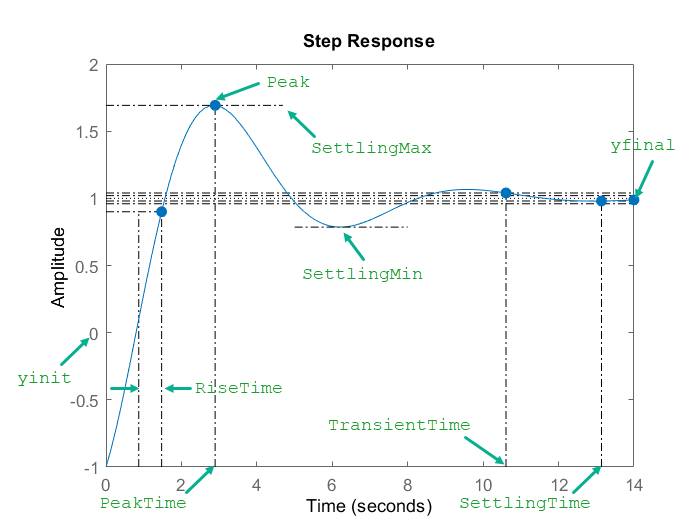example

````S = stepinfo(sys)` computes the step-response characteristics for a dynamic system model `sys`. This syntax uses yinit = 0 and yfinal = steady-state value for computing the characteristics that depend on these values.```
````S = stepinfo(y,t)` computes step-response characteristics from an array of step-response data `y` and a corresponding time vector `t`. For SISO system responses, `y` is a vector with the same number of entries as `t`. For MIMO response data, `y` is an array containing the responses of each I/O channel. This syntax uses yinit = 0 and the last value in `y` (or the last value in each channel's corresponding response data) as yfinal.```

example

````S = stepinfo(y,t,yfinal)` computes step-response characteristics relative to the steady-state value `yfinal`. This syntax is useful when you know that the expected steady-state system response differs from the last value in `y` for reasons such as measurement noise. This syntax uses yinit = 0.For SISO responses, `t` and `y` are vectors with the same length `NS`. For systems with `NU` inputs and `NY` outputs, you can specify `y` as an `NS`-by-`NY`-by-`NU` array (see `step`) and `yfinal` as an `NY`-by-`NU` array. `stepinfo` then returns a `NY`-by-`NU` structure array `S` of response characteristics corresponding to each I/O pair.```

example

````S = stepinfo(y,t,yfinal,yinit)` computes step-response characteristics relative to the response initial value `yinit`. This syntax is useful when your `y` data has an initial offset; that is, `y` is nonzero before the step occurs.For SISO responses, `t` and `y` are vectors with the same length `NS`. For systems with `NU` inputs and `NY` outputs, you can specify `y` as an `NS`-by-`NY`-by-`NU` array and `yinit` as an `NY`-by-`NU` array. `stepinfo` then returns a `NY`-by-`NU` structure array `S` of response characteristics corresponding to each I/O pair.```

example

````S = stepinfo(___,'SettlingTimeThreshold',ST)` lets you specify the threshold `ST` used in the definition of settling and transient times. The default value is `ST = 0.02` (2%). You can use this syntax with any of the previous input-argument combinations. ```

example

````S = stepinfo(___,'RiseTimeLimits',RT)` lets you specify the lower and upper thresholds used in the definition of rise time. By default, the rise time is the time the response takes to rise from 10% to 90% of the way from the initial value to the steady-state value (```RT = [0.1 0.9]```). The upper threshold `RT(2)` is also used to calculate `SettlingMin` and `SettlingMax`. These values are the minimum and maximum values of the response occurring after the response reaches the upper threshold. You can use this syntax with any of the previous input-argument combinations. ```

## Examples

collapse all

Compute step-response characteristics, such as rise time, settling time, and overshoot, for a dynamic system model. For this example, use a continuous-time transfer function:

`$sys=\frac{{s}^{2}+5s+5}{{s}^{4}+1.65{s}^{3}+5{s}^{2}+6.5s+2}$`

Create the transfer function and examine its step response.

```sys = tf([1 5 5],[1 1.65 5 6.5 2]); step(sys)```The plot shows that the response rises in a few seconds, and then rings down to a steady-state value of about 2.5. Compute the characteristics of this response using `stepinfo`.

`S = stepinfo(sys)`
```S = struct with fields: RiseTime: 3.8456 TransientTime: 27.9762 SettlingTime: 27.9762 SettlingMin: 2.0689 SettlingMax: 2.6873 Overshoot: 7.4915 Undershoot: 0 Peak: 2.6873 PeakTime: 8.0530 ```

Here, the function uses ${\mathit{y}}_{\mathrm{init}}$= 0 to compute characteristics for the dynamic system model `sys`.

By default, the settling time is the time it takes for the error to stay below 2% of $|{\mathit{y}}_{\mathrm{init}}-{\mathit{y}}_{\mathit{final}}|$. The result `S.SettlingTime` shows that for `sys`, this condition occurs after about 28 seconds. The default definition of rise time is the time it takes for the response to go from 10% to 90% of the way from ${\mathit{y}}_{\mathrm{init}}$= 0 to ${\mathit{y}}_{\mathrm{final}}$. `S.RiseTime` shows that for `sys`, this rise occurs in less than 4 seconds. The maximum overshoot is returned in `S.Overshoot`. For this system, the peak value `S.Peak`, which occurs at the time `S.PeakTime`, overshoots by about 7.5% of the steady-state value.

For a MIMO system, `stepinfo` returns a structure array in which each entry contains the response characteristics of the corresponding I/O channel of the system. For this example, use a two-output, two-input discrete-time system. Compute the step-response characteristics.

```A = [0.68 -0.34; 0.34 0.68]; B = [0.18 -0.05; 0.04 0.11]; C = [0 -1.53; -1.12 -1.10]; D = [0 0; 0.06 -0.37]; sys = ss(A,B,C,D,0.2); S = stepinfo(sys)```
```S=2×2 struct array with fields: RiseTime TransientTime SettlingTime SettlingMin SettlingMax Overshoot Undershoot Peak PeakTime ```

Access the response characteristics for a particular I/0 channel by indexing into `S`. For instance, examine the response characteristics for the response from the first input to the second output of `sys`, corresponding to `S(2,1)`.

`S(2,1)`
```ans = struct with fields: RiseTime: 0.4000 TransientTime: 2.8000 SettlingTime: 3 SettlingMin: -0.6724 SettlingMax: -0.5188 Overshoot: 24.6476 Undershoot: 11.1224 Peak: 0.6724 PeakTime: 1 ```

To access a particular value, use dot notation. For instance, extract the rise time of the (2,1) channel.

`rt21 = S(2,1).RiseTime`
```rt21 = 0.4000 ```

You can use `SettlingTimeThreshold` and `RiseTimeThreshold` to change the default percentage for settling and rise times, respectively, as described in the Algorithms section. For this example, use the system given by:

`$\mathit{sys}=\frac{{\mathit{s}}^{2}+5\mathit{s}+5}{{\mathit{s}}^{4}+1.65{\mathit{s}}^{3}+6.5\mathit{s}+2}$`

Create the transfer function.

`sys = tf([1 5 5],[1 1.65 5 6.5 2]);`

Compute the time it takes for the error in the response of `sys` to stay below 0.5% of the gap $|{\mathit{y}}_{\mathrm{final}}-{\mathit{y}}_{\mathrm{init}}|$. To do so, set `SettlingTimeThreshold` to 0.5%, or 0.005.

```S1 = stepinfo(sys,'SettlingTimeThreshold',0.005); st1 = S1.SettlingTime```
```st1 = 46.1325 ```

Compute the time it takes the response of `sys` to rise from 5% to 95% of the way from ${\mathit{y}}_{\mathrm{init}}$ to ${\mathit{y}}_{\mathrm{final}}$. To do so, set `RiseTimeThreshold` to a vector containing those bounds.

```S2 = stepinfo(sys,'RiseTimeThreshold',[0.05 0.95]); rt2 = S2.RiseTime```
```rt2 = 4.1690 ```

You can define percentages for both settling time and rise time in the same computation.

`S3 = stepinfo(sys,'SettlingTimeThreshold',0.005,'RiseTimeThreshold',[0.05 0.95])`
```S3 = struct with fields: RiseTime: 4.1690 TransientTime: 46.1325 SettlingTime: 46.1325 SettlingMin: 2.0689 SettlingMax: 2.6873 Overshoot: 7.4915 Undershoot: 0 Peak: 2.6873 PeakTime: 8.0530 ```

You can extract step-response characteristics from step-response data even if you do not have a model of your system. For instance, suppose you have measured the response of your system to a step input and saved the resulting response data in a vector `y` of response values at the times stored in another vector `t`. Load the response data and examine it.

```load StepInfoData t y plot(t,y)```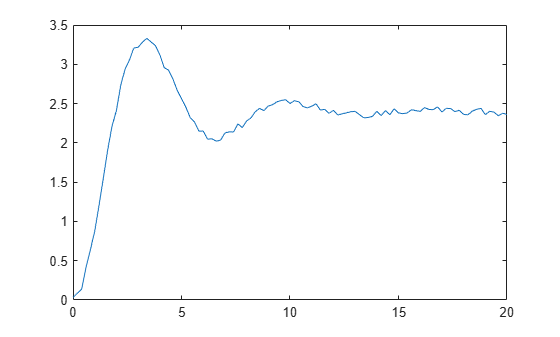Compute step-response characteristics from this response data using `stepinfo`. If you do not specify the steady-state response value `yfinal`, then `stepinfo` assumes that the last value in the response vector `y` is the steady-state response. Because the data has some noise, the last value in `y` is likely not the true steady-state response value. When you know what the steady-state value should be, you can provide it to `stepinfo`. For this example, suppose that the steady-state response is 2.4.

`S1 = stepinfo(y,t,2.4)`
```S1 = struct with fields: RiseTime: 1.2897 TransientTime: 19.6478 SettlingTime: 19.6439 SettlingMin: 2.0219 SettlingMax: 3.3302 Overshoot: 38.7575 Undershoot: 0 Peak: 3.3302 PeakTime: 3.4000 ```

Because of the noise in the data, the default definition of the settling time is too stringent, resulting in an arbitrary value of almost 20 seconds. To allow for the noise, increase the settling-time threshold from the default 2% to 5%.

`S2 = stepinfo(y,t,2.4,'SettlingTimeThreshold',0.05)`
```S2 = struct with fields: RiseTime: 1.2897 TransientTime: 10.4201 SettlingTime: 10.4149 SettlingMin: 2.0219 SettlingMax: 3.3302 Overshoot: 38.7575 Undershoot: 0 Peak: 3.3302 PeakTime: 3.4000 ```

Settling time and transient time are equal when the peak error ${\mathit{e}}_{\mathrm{max}}$ is equal to the gap $|{\mathit{y}}_{\mathrm{final}}-{\mathit{y}}_{\mathrm{init}}|$ (see Algorithms), which is the case for models with no undershoot or feedthrough and with less than 100% overshoot. They tend to differ for models with feedthrough, zeros at the origin, unstable zeros (undershoot), or large overshoot.

Consider the following models.

```s = tf('s'); sys1 = 1+tf(1,[1 1]); % feedthrough sys2 = tf([1 0],[1 1]); % zero at the origin sys3 = tf([-3 1],[1 2 1]); % non-minimum phase with undershoot sys4 = (s/0.5 + 1)/(s^2 + 0.2*s + 1); % large overshoot step(sys1,sys2,sys3,sys4) grid on legend('Feedthrough','Zero at origin','Non-minimum phase with undershoot','Large overshoot')```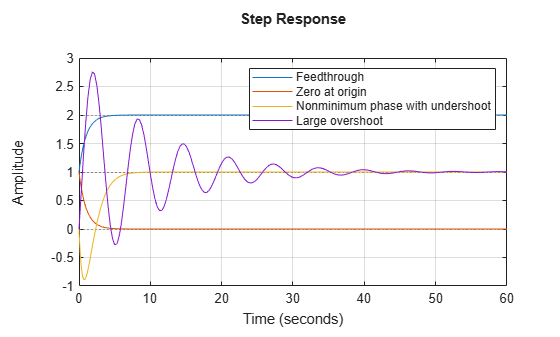Compute the step-response characteristics.

`S1 = stepinfo(sys1)`
```S1 = struct with fields: RiseTime: 1.6095 TransientTime: 3.9121 SettlingTime: 3.2190 SettlingMin: 1.8005 SettlingMax: 1.9993 Overshoot: 0 Undershoot: 0 Peak: 1.9993 PeakTime: 7.3222 ```
`S2 = stepinfo(sys2)`
```S2 = struct with fields: RiseTime: 0 TransientTime: 3.9121 SettlingTime: NaN SettlingMin: 6.6069e-04 SettlingMax: 1 Overshoot: Inf Undershoot: 0 Peak: 1 PeakTime: 0 ```
`S3 = stepinfo(sys3)`
```S3 = struct with fields: RiseTime: 2.9198 TransientTime: 6.5839 SettlingTime: 7.3229 SettlingMin: 0.9004 SettlingMax: 0.9991 Overshoot: 0 Undershoot: 88.9466 Peak: 0.9991 PeakTime: 10.7900 ```
`S4 = stepinfo(sys4)`
```S4 = struct with fields: RiseTime: 0.3896 TransientTime: 40.3317 SettlingTime: 46.5052 SettlingMin: -0.2796 SettlingMax: 2.7571 Overshoot: 175.7137 Undershoot: 27.9629 Peak: 2.7571 PeakTime: 1.8850 ```

Examine the plots and characteristics. For these models, the settling time and transient time differ because the peak error exceeds the gap between the initial and the final value. For models such as `sys2`, the settling time is returned as `NaN` because the steady-state value is zero.

In this example, you compute the step-response characteristics from step-response data that has an initial offset. This means that the value of the response data is nonzero before the step occurs.

Load the step-response data and examine the plot.

```load stepDataOffset.mat plot(stepOffset.Time,stepOffset.Data)```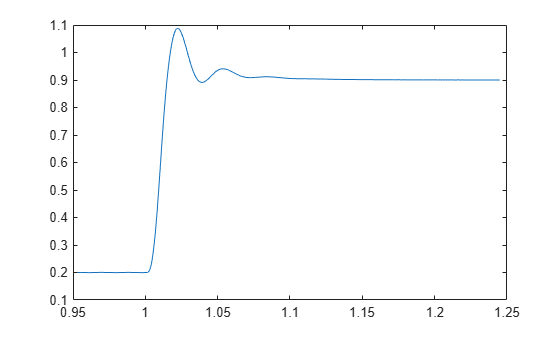If you do not specify `yfinal` and `yinit`, then `stepinfo` assumes that `yfinal` is the last value in the response vector `y` and `yinit` is zero. When you know what the steady-state and initial values are, you can provide them to `stepinfo`. Here, the steady state of the response `yfinal` is 0.9 and the initial offset `yinit` is 0.2.

Compute step-response characteristics from this response data.

`S = stepinfo(stepOffset.Data,stepOffset.Time,0.9,0.2)`
```S = struct with fields: RiseTime: 0.0084 TransientTime: 1.0662 SettlingTime: 1.0662 SettlingMin: 0.8461 SettlingMax: 1.0878 Overshoot: 26.8259 Undershoot: 0.0429 Peak: 0.8878 PeakTime: 1.0225 ```

Here, the peak value of this response is 0.8878 because `stepinfo` measures the maximum deviation from `yinit`.

## Input Arguments

collapse all

Dynamic system, specified as a SISO or MIMO dynamic system model. Dynamic systems that you can use include:

• Continuous-time or discrete-time numeric LTI models, such as `tf`, `zpk`, or `ss` models.

• Generalized or uncertain LTI models such as `genss` or `uss` (Robust Control Toolbox) models. (Using uncertain models requires Robust Control Toolbox™ software.) For generalized models, `stepinfo` computes the step-response characteristics using the current value of tunable blocks and the nominal value of uncertain blocks.

• Identified LTI models, such as `idtf` (System Identification Toolbox), `idss` (System Identification Toolbox), or `idproc` (System Identification Toolbox) models. (Using identified models requires System Identification Toolbox™ software.)

Step-response data, specified as one of the following:

• For SISO response data, a vector of length `Ns`, where `Ns` is the number of samples in the response data

• For MIMO response data, an `Ns`-by-`Ny`-by-`Nu` array, where `Ny` is the number of system outputs and `Nu` is the number of system inputs

Time vector corresponding to the response data in `y`, specified as a vector of length `Ns`.

Steady-state value, specified as a scalar or an array.

• For SISO response data, specify a scalar value.

• For MIMO response data, specify an `Ny`-by-`Nu` array, where each entry provides the steady-state response value for the corresponding system channel.

If you do not provide `yfinal`, then `stepinfo` uses the last value in the corresponding channel of `y` as the steady-state response value.

This argument is only supported when you provide step-response data as an input. For a dynamic system model `sys` as an input, `stepinfo` uses yfinal = steady-state value to compute the characteristics that depend on this value.

Value of `y` before the step occurs, specified as a scalar or an array.

• For SISO response data, specify a scalar value.

• For MIMO response data, specify an `Ny`-by-`Nu` array, where each entry provides the response initial value for the corresponding system channel.

If you do not provide `yinit`, then `stepinfo` uses zero as the response initial value.

The response y(0) at t = 0 is equal to yinit for systems without feedthrough. However, the two quantities differ in the presence of feedthrough because of the discontinuity at t = 0.

For example, the following figure shows the step response of a system with feedthrough `sys = tf([-1 0.2 1],[1 0.7 1])`.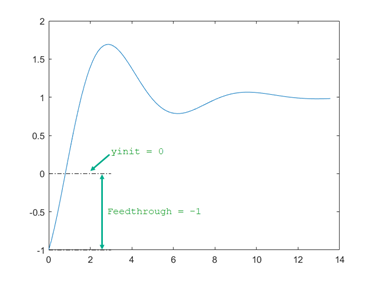Here, yinit is zero and the feedthrough value is –1.

This argument is only supported when you provide step-response data as an input. For a dynamic system model `sys` as an input, `stepinfo` uses yinit = 0 to compute the characteristics that depend on this value.

Threshold for defining settling and transient times, specified as a scalar value between 0 and 1. To change the default settling and transient time definitions (see Algorithms), set `ST` to a different value. For instance, to measure when the error falls below 5%, set `ST` to 0.05.

Threshold for defining rise time, specified as a 2-element row vector of nondescending values between 0 and 1. To change the default rise time definition (see Algorithms), set `RT` to a different value. For instance, to define the rise time as the time it takes for the response to rise from 5% to 95% from the initial value to the steady-state value, set `RT` to `[0.05 0.95]`.

## Output Arguments

collapse all

Step-response characteristics, returned as a structure containing the fields:

• `RiseTime`

• `TransientTime`

• `SettlingTime`

• `SettlingMin`

• `SettlingMax`

• `Overshoot`

• `Undershoot`

• `Peak`

• `PeakTime`

For more information on how `stepinfo` defines these characteristics, see Algorithms.

For MIMO models or responses data, `S` is a structure array in which each entry contains the step-response characteristics of the corresponding I/O channel. For instance, if you provide a 3-input, 3-output model or an array of response data, then `S(2,3)` contains the characteristics of the response from the third input to the second output. For an example, see Step-Response Characteristics of MIMO System.

If `sys` is unstable, then all step-response characteristics are `NaN`, except for `Peak` and `PeakTime`, which are `Inf`.

## Algorithms

For a step response y(t), `stepinfo` computes characteristics relative to yinit and yfinal. For a dynamic system model `sys`, `stepinfo` uses yinit = 0 and yfinal = steady-state value.

This table shows how `stepinfo` computes each characteristic.

Step-Response CharacteristicDescription
`RiseTime`Time it takes for the response to rise from 10% to 90% of the way from yinit to yfinal
`TransientTime`

The first time T such that the error |y(t) – yfinal| ≤ SettlingTimeThreshold × emax for tT, where emax is the maximum error |y(t) – yfinal| for t ≥ 0.

By default, SettlingTimeThreshold = 0.02 (2% of the peak error). Transient time measures how quickly the transient dynamics die off.

`SettlingTime`

The first time T such that the error |y(t) – yfinal| ≤ SettlingTimeThreshold × |yfinalyinit| for tT.

By default, `SettlingTime` measures the time it takes for the error to stay below 2% of |yfinalyinit|.

`SettlingMin`Minimum value of y(t) once the response has risen
`SettlingMax`Maximum value of y(t) once the response has risen
`Overshoot`Percentage overshoot. Relative to the normalized response ynorm(t) = (y(t) – yinit)/(yfinalyinit), the overshoot is the larger of zero and 100 × max(ynorm(t) – 1).
`Undershoot`Percentage undershoot. Relative to the normalized response ynorm(t), the undershoot is the smaller of zero and –100 × max(ynorm(t) – 1).
`Peak`Peak value of |y(t) – yinit|
`PeakTime`Time at which the peak value occurs

## Version History

Introduced in R2006a

expand all